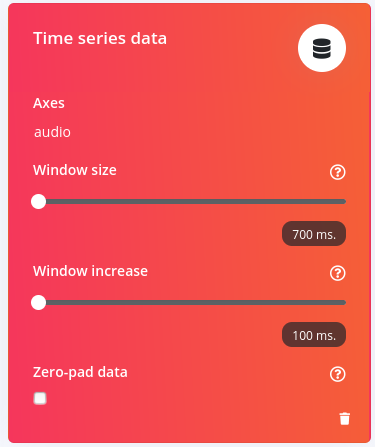# Help understanding mfcc's parameters

Hi, I am looking for help in trying to understand some of the parameters of the MFCC process block.
Here are the parameters I chose in this example.

I

First of all let me explain what I understood :

1. first each sample of audio data that is fed to the block is 0.7s long (i.e 11200samples working at 16khz sample rate)
2. Then the 0.7s long sample is sliced into 35 (0.7/0.02=35) 0.02s audio frames which are 320samples (0.02*16000) long
3. Then each frame is windowed and the pre emphasis is applied
4. Then the fft and the rest is applied.

From this starting point I have a few questions to ask :

• First what kind of window is applied each time on the audio (is it hamming ?)?
• Here the frame length in samples (320) is bigger than the fft length (256). What does that mean ? Is the end, the beginning or both chopped before performing the fft.
• What does the shift value symbolizes in the pre-emphasis section ?
• For the filtering, what kind of formula is used, HTK, Slaney, other ?
• Is the signal normalized at any point before being fed to the neural network ?

Thank you very much for any answer !

Here is the settings of the “Time series data”Thanks for the questions @clem! Answers inline with questions:

• First what kind of window is applied each time on the audio (is it hamming ?)?
-Rectangular

• Here the frame length in samples (320) is bigger than the fft length (256). What does that mean ? Is the end, the beginning or both chopped before performing the fft.
-The end is chopped off. If fft_size is larger than frame length, then we zero pad.

• What does the shift value symbolizes in the pre-emphasis section ?
-The pre emphasis function is this formula: y[n] = x[n] - coefficient*x[n-shift]. Which is a first order, high pass filter (essentially, you’re attenuating the low frequency just a little bit so the energy is more balanced between low and high frequencies). When you adjust shift, you’re essentially moving the zero around on the Z plane

• For the filtering, what kind of formula is used, HTK, Slaney, other ?
I would need more detail to answer this question fully, but I believe the algorithms you’re referring to play with the spacing and frequencies of the filters. We space the filters based on the original MFCC work in Davis and Mermelstein, 1980. For more details, checkout the code from our exporter, edge-impulse-sdk/dsp/speechpy/feature.hpp, the function “filterbanks”

• Is the signal normalized at any point before being fed to the neural network ?

• Yes, the final step is to normalize the output of the MFCC block for each sample ( ie in your case, the features output by processing 0.7s or 11200 samples of data ), into the domain [0,1.0], before being fed to the NN. (This is in addition to the frame level mean and variance normalization that happens within the DSP block, typical to MFCC implementations)

Also, just for the reference of anyone else reading this post, additional info can be found in our doc for this block:

1 Like

Hi, thank you this clears up a lot !
I still have a few questions to ask• I’m unsure of my understanding of the normalization part. As you can see on the bottom right of the image, the processed features can have values above 1 (and on other examples above 4 I think). But what I understood from your answer is that each block have values in [0, 1]. Where is my mistake ?

• The other point which I am unsure of is the processed features representation :
This is a 2D array stored as 1D. in my case of length 455 (13*35)
Does that mean that the 13 first values corresponds to the 13 coefficients of the first mfcc column ?
That’s what I assumed in the following python code but I get different renders when plotting the array and viewing the representation on the website. (I think there is some kind of offset introduced somwhere that shifts the values ?)

@clem Just a note that we’re still looking into these two things. Thanks for pointing them out

@clem Thanks for pointing out the graph vs features. A fix just hit production, try it now and it should be a better match.

As for normalization, I stand corrected. I was looking at the wrong block of code. We don’t normalize to [0,1]. However, there is some mean and variance normalization done, which is typical of MFCC implementations.

3 Likes